V.6 No 1 13 On excited state of orbital electronWith such relation of frequencies, in full accordance with Bohr’s theory, resonances also will take place and the pattern of trajectories of excited electron will remain on the whole. As an example, in Fig. 13 we show the diagrams for several relations of frequencies.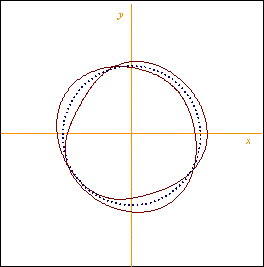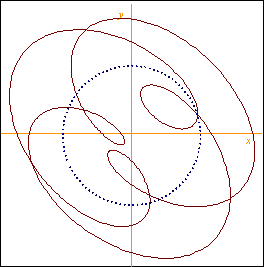a) E0 = 6,16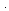10 -4 V/m                                             b) E0 = 4,1810 -3 V/mE = (5/2)e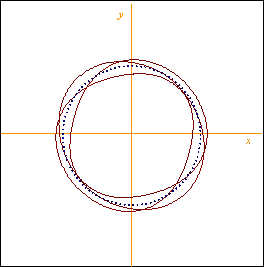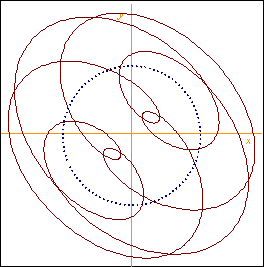a) E0 = 5,6010 -4 V/m                                             b) E0 = 3,9710 -3 V/mE = (7/3)e
 Fig. 13. Trajectories of the excited electron’s motion at the affecting force frequency exceeding the natural frequency of orbital electron in a non-integer number of times: a) in a weak field, b) in a strong field
 The only distinction of non-integer resonances is, the intensity of electron’s radiation at these frequencies abruptly falls. Just so with the growing number of series it is possible to observe series with less pressure, i.e. with less mutual affection of hydrogen atoms that blurs little-intensive spectral lines. The resonance characteristics of the studied atomic system remain also at the natural frequency of electron exceeding the frequency of external field. In Fig. 14 we see related diagrams.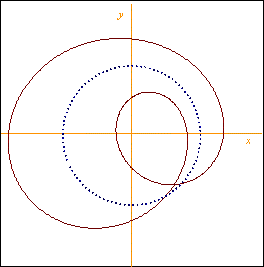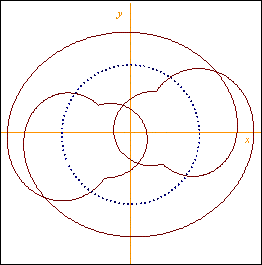a)E = (1/2)e                                             b)E = (1/3)e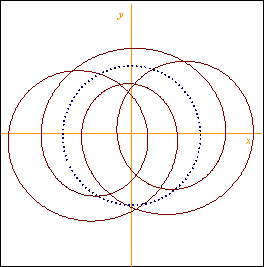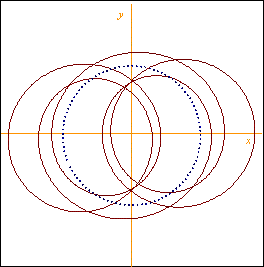c)E = (1/4)e                                             d)E = (1/5)e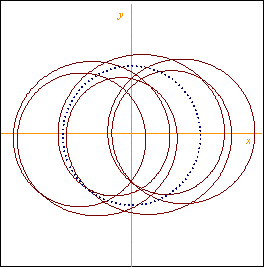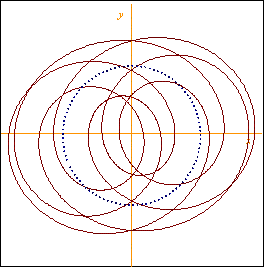e)E = (1/6)e                                             f)E = (3/7)e
 Fig. 14. Trajectories of the excited electron’s motion at the natural frequency exceeding the frequency of orbital electron affecting force: a) in a weak field, b) in a strong field
 As we see, in this case the return loops are absent and the number of electron’s turns per one full period is in a strong correspondence with the coefficient of exceeding of natural frequency over the frequency of external field. But in this case also the quantified levels of energy are absent as such, while the resonance pattern of excitation remains. From these diagrams we can follow the phenomenology, how the absorption bands arise. Because the electron’s reaction is opposite to the external excitation, the atom system under the external field affection becomes a secondary radiator that compensates the external field. The degree of this compensation depends on many factors. First of all it relates to the delay phases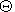x andy in (80). As is simple to see, these phases depend on time, and the oscillation amplitude is inconstant, too. The utmost counter-radiation is achieved with the strongly antiphase radiation secondary to that inducting. Secondly, the degree of external field compensation lessens with larger ratio of the frequencies, in full accordance with the effectiveness of dipole radiation. And thirdly, the electron’s excitation amplitude is maximal just for the main resonanceE =e , which is well seen in the diagram, Fig. 15, where we show the regularity of the exciting field amplitude E0 against the ratio of excitation frequency to the natural frequency of electron, which leads us to the equal maximal oscillation amplitude.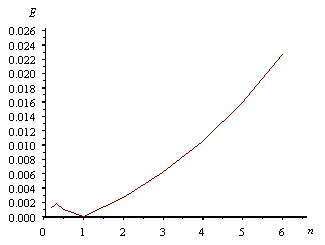Fig. 15. The amplitude of exciting field E0 against the ratio of excitation frequency to the natural frequency of electron n, at equal amplitudes of electron’s excitation
 We would add, the case of excitation and radiation resulting from atom’s interaction with free electrons that we see, e.g., in the arc processes is similar to the above, with the stipulation that the field of free electron interacting with the orbital electron is described by the continual spectrum of frequencies of which the system of atom selects those corresponding to its resonance frequencies. With it in the modelling system (66), the related spectral characteristics of the field of free electron interacting with the atom will be present as an exciting field. Thus, we see, the real pattern of orbital electron’s excitation basically differs from the model on whose basis the quantum-mechanical methods have been built. The resonanceness of atom’s system is determined not by quantified levels of energy but by natural frequency of the orbital electron matched with the frequency of external field. And this is well seen already on the linear model, despite all its incompleteness. If we additionally account the nonlinearity of processes which we can perform in the way shown in , it will improve the trajectories. But in this case also, as we showed, the first harmonic will correspond to the solutions which we yielded for the linear model.

Contents: / 1 / 2 / 3 / 4 / 5 / 6 / 7 / 8 / 9 / 10 / 11 / 12 / 13 / 14 / 15 /